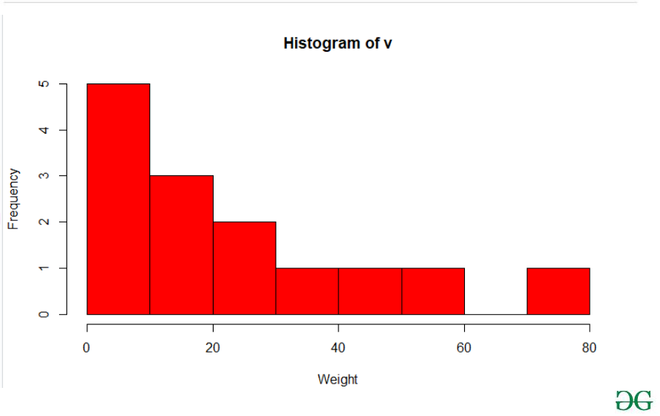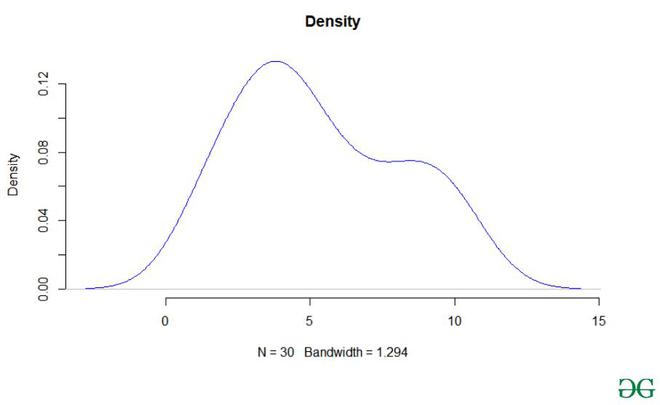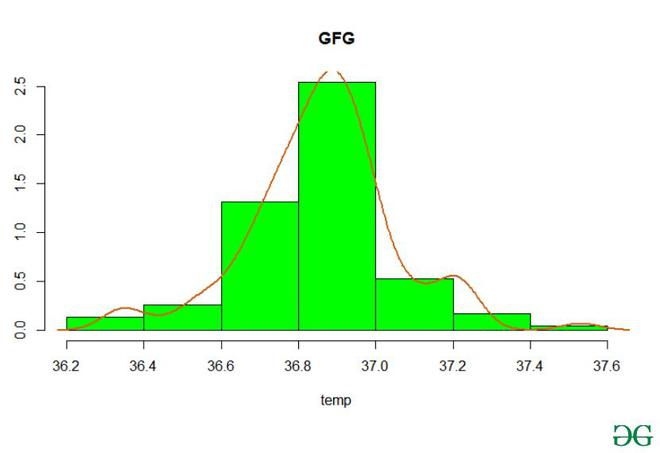Open in App
Not now

# Histograms and Density Plots in R

• Last Updated : 25 Feb, 2021

A histogram is a graphical representation that organizes a group of data points into user-specified ranges and an approximate representation of the distribution of numerical data.

In R language the histogram is built with the use of hist() function.

Syntax: hist(v,main,xlab,xlim,ylim,breaks,col,border)

Parameters:

• v:- It is a vector containing numeric values used in the histogram.
• main:-It indicates the title of the chart.
• col:- It is used to set the color of the bars.
• border:-It is used to set the border color of each bar.
• xlab:-It is used to give a description of the x-axis.
• xlim:-It is used to specify the range of values on the x-axis.
• ylim:-It is used to specify the range of values on the y-axis.
• breaks:-It is used to mention the width of each bar.

Return: It will return the histogram.

Example:-

## R

 `v <-  ``c``(5,9,13,2,50,20,59,36,23,2,8,27,72,14)`` ` `hist``(v,xlab = ``"Weight"``,col = ``"red"``,border = ``"black"``)`

Output:A density plot is a representation of the distribution of a numeric variable that uses a kernel density estimate to show the probability density function of the variable. In R Language we use the density() function which helps to compute kernel density estimates. And further with its return value, is used to build the final density plot.

Syntax: density(x)

Parameters:

• x: the data from which the estimate is to be computed

Returns:

It will return the kernel density.

Example:

## R

 `library``(readxl)``library``(ggplot2)`` ` `Salary_Data <- ``read_excel``(``"Salary_Data.xls"``)`` ` `den <- ``density``(Salary_Data\$YearsExperience)`` ` `plot``(den, frame = ``FALSE``, col = ``"blue"``,main = ``"Density plot"``)`

Output:We can also create a histogram and a density plot in the same frame.

Example:

## R

 `hist``(beaver1\$temp, ``     ``col=``"green"``,``     ``border=``"black"``,``     ``prob = ``TRUE``,``     ``xlab = ``"temp"``,``     ``main = ``"GFG"``)`` ` `lines``(``density``(beaver1\$temp),``      ``lwd = 2,``      ``col = ``"chocolate3"``)`

Output:My Personal Notes arrow_drop_up